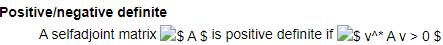Re: [eigen] Using Eigen decompositions with ceres autodiff Jet data type.
• To: eigen@xxxxxxxxxxxxxxxxxxx
• Subject: Re: [eigen] Using Eigen decompositions with ceres autodiff Jet data type.
• From: Patrik Huber <patrikhuber@xxxxxxxxx>
• Date: Mon, 1 Jun 2020 18:33:01 +0100
• Dkim-signature: v=1; a=rsa-sha256; c=relaxed/relaxed; d=gmail.com; s=20161025; h=mime-version:references:in-reply-to:from:date:message-id:subject:to; bh=KAg3a6BD1AOjewf68H5dpGYWwpNZvR/cIdTF9Cx/I2Q=; b=slxgnKH9NvH/qsYY7EQldt6940T0zjdoXJUTgvA6/RMEX1wQvtfB7ALSTkQngHI5uP oLBhwr/hREIJL7vmpQMZSbcbfWckpHJucDYdN9wfH36NghDutPsxtNXyxpHOztrArVbU EaUv8O5VBvL/mZDM3Y3IkJbAm/KC5KhjfF3Q2rBm41bfT4E7gKG/iEyiGzuy06Y/lUUy g2npX3+PnrEJDZyIZS0ADO7ZjxddYl9NNbTPIZQaVCEc2MVKYI8ZfWYzR6zG9+6L9lnG l/UgrtsaGRCYIvGAY3xYjGAEFj8CeM+Pwg/G38DccSJuXE7ImZtqkpLqDtpyHzW9p1uX 9yQA==

Hi Rasmus,

This is slightly off-topic to this thread here, but it would be great if you added your COD to the list/table of decompositions in Eigen: https://eigen.tuxfamily.org/dox/group__TopicLinearAlgebraDecompositions.html
First, it would make it easier for people to find, and second, it would also help a lot to see on that page how the algorithm compares to the others, to be able to choose it appropriately.

Unrelated: @All/Maintainers: It seems like lots (all) of the images on the documentation website are broken? At least for me. E.g.:Best wishes,
Patrik

On Mon, 1 Jun 2020 at 17:59, Rasmus Munk Larsen <rmlarsen@xxxxxxxxxx> wrote:
Hi Oleg and Sameer,

A faster option than SVD, but more robust than QR (since it also handles the under-determined case) is the complete orthogonal decomposition that I implemented in Eigen a few years ago.

https://eigen.tuxfamily.org/dox/classEigen_1_1CompleteOrthogonalDecomposition.html

(Looks like the docstring is broken - oops!)

It appears to also be available in the 3.3 branch: https://gitlab.com/libeigen/eigen/-/blob/3.3/Eigen/src/QR/CompleteOrthogonalDecomposition.h

Rasmus

On Mon, Jun 1, 2020 at 6:57 AM Sameer Agarwal <sameeragarwal@xxxxxxxxxx> wrote:
Oleg,
Two ideas:

1. You may have an easier time using QR factorization instead of SVD to solve your least squares problem.
2.  But you can do better, instead of trying to solve linear least squares problem involving a matrix of Jets, you are better off, solving the linear least squares problem on the scalars, and then using the implicit function theorem to compute the derivative w.r.t the parameters and then applying the chain rule.

the solution satisfies the equation

A'A x - A'b = 0.

solve this equation to get the optimal value of x, and then compute the jacobian of this equation w.r.t A, b and x. and apply the implicit theorem.

Sameer

On Mon, Jun 1, 2020 at 4:46 AM Oleg Shirokobrod <oleg.shirokobrod@xxxxxxxxx> wrote:
Hi list, I am using Eigen 3.3.7 release with ceres solver 1.14.0 with autodiff Jet data type and I have some problems. I need to solve linear least square subproblem within variable projection algorithm, namely I need to solve LLS equation
A(p)*x = b
Where matrix A(p) depends on nonlinear parameters p:
x(p) = pseudo-inverse(A(p))*b;
x(p) will be optimized in nonlinear least squares fitting, so I need Jcobian. Rhs b is measured vector of doubles, e.g. VectorXd. In order to use ceres's autodiff p must be of Jet type. Ceres provides corresponding traits for binary operations

#if EIGEN_VERSION_AT_LEAST(3, 3, 0)
// Specifying the return type of binary operations between Jets and scalar types
// allows you to perform matrix/array operations with Eigen matrices and arrays
// such as addition, subtraction, multiplication, and division where one Eigen
// matrix/array is of type Jet and the other is a scalar type. This improves
// performance by using the optimized scalar-to-Jet binary operations but
// is only available on Eigen versions >= 3.3
template <typename BinaryOp, typename T, int N>
struct ScalarBinaryOpTraits<ceres::Jet<T, N>, T, BinaryOp> {
typedef ceres::Jet<T, N> ReturnType;
};
template <typename BinaryOp, typename T, int N>
struct ScalarBinaryOpTraits<T, ceres::Jet<T, N>, BinaryOp> {
typedef ceres::Jet<T, N> ReturnType;
};
#endif  // EIGEN_VERSION_AT_LEAST(3, 3, 0)

There two problems.
1. Small problem. In a function "RealScalar threshold() const" in SCDbase.h I have to replace "return m_usePrescribedThreshold ? m_prescribedThreshold
: diagSize* NumTraits<Scalar>::epsilon();" with "return m_usePrescribedThreshold ? m_prescribedThreshold
: Scalar(diagSize)* NumTraits<Scalar>::epsilon();"
This fix is similar Gael's fix of Bug 1403
2. It is less trivial. I expect that x(p) = pseudo-inverse(A(p))*b; is vector of Jet. And it is actually true for e.g SVD decompoazition
x(p) = VSU^T * b.
But if I use
JcobySVD<Matrix<Jet<double, 2>, Dynamic, Dynamic>> svd(A);
x(p) = svd.solve(b),
I got error message.
Here code for reproducing the error

// test_svd_jet.cpp
#include <ceres/jet.h>
using ceres::Jet;

int test_svd_jet()
{
typedef Matrix<Jet<double, 2>, Dynamic, Dynamic> Mat;
typedef Matrix<Jet<double, 2>, Dynamic, 1> Vec;
Mat A = MatrixXd::Random(3, 2).cast <Jet<double, 2>>();
VectorXd b = VectorXd::Random(3);
JacobiSVD<Mat> svd(A, ComputeThinU | ComputeThinV);
int l_rank = svd.rank();
Vec c = svd.matrixV().leftCols(l_rank)
* svd.matrixU().leftCols(l_rank).adjoint() * b; // *
Vec c1 = svd.solve(b.cast<Jet<double, 2>>()); // **
Vec c2 = svd.solve(b); // ***
return 0;
}
// End test_svd_jet.cpp

// * and // ** work fine an give the same results. // *** fails with VS 2019 error message
Eigen\src\Core\functors\AssignmentFunctors.h(24,1):
error C2679: binary '=': no operator found which takes
a right-hand operand of type 'const SrcScalar'
(or there is no acceptable conversion)
The error points to line //***. I thing that solution is of type VectorXd instead of Vec and there is problem with assignment of double to Jet. Derivatives are lost either. It should work similar to complex type. If A is complex matrix and b is real vector, x must be complex. There is something wrong with Type deduction in SVD or QR decomposition.

Do you have any idea of how to fix it.

Best regards,

Oleg Shirokobrod

 Mail converted by MHonArc 2.6.19+ http://listengine.tuxfamily.org/